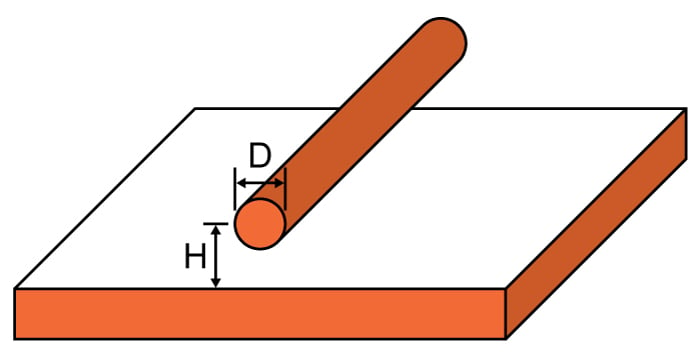# Wire Over Plane Inductance Calculator

## This calculator helps you compute the inductance of a wire-over-plane setup.

H

### Overview

A wire over a ground plane also exhibits inductance and may or may not contribute to the circuit board or transmission line's performance. This calculator helps you find out how much inductance a wire over a plane represents given the diameter and length of the wire, and its height above the plane.

To use this tool, enter the wire diameter, wire length, height of the wire above the plane and the relative permeability between the wire and the plane. If only air is present between the wire and the plane, a value of 1 is a good approximation. Note that you can select measurement units (mm, cm, mil, um (micrometre) and inch), for the length, diameter and height.### Equation

$$L_{wop}\approx \frac{\mu _{o}\mu _{r}L}{2\pi}\cosh^{-1}\left ( \frac{H}{D} \right )$$

Where:

$$L_{wop}$$ = inductance of the wire over plane in henries (H)

$$\mu_{0}$$ = permeability of free space = 4π×10−7

$$\mu_{r}$$ = relative permeability

$$D$$ = diameter of the wire

$$H$$ = height of the wire above the plane

$$L$$ = length of the wire

### Applications

Calculating the inductance of a wire-over-a-plane setup is significant, as such a setup is a common occurrence in transmission lines and printed circuit boards. In radio frequency applications, any inductance may or may not contribute to the performance of the line or the circuit. An inductance, at the right frequency, can filter, attenuate, or even radiate signals unintentionally.